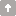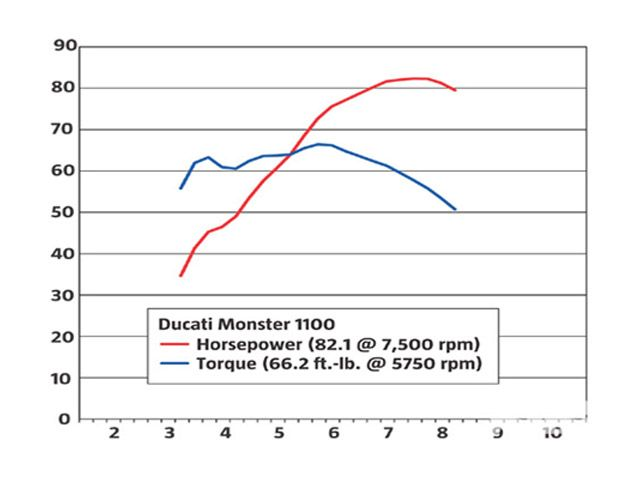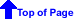# Debunking Horsepower and Torque Myths

Torque is all that counts! Ive got more horsepower, thats what counts! Ive got good low-end power, thats what counts!

There is a lot of discussion among motor enthusiasts regarding horsepower and torque - resulting in heated conversations containing misconceptions and errors. This article explains how torque and horsepower are related, and exposes some of the common misconceptions.

## What is torque, and what is horsepower?

First, what is torque? Torque is a twisting force on an object. If you have ever used a torque wrench, you can relate to this. The torque wrench reads how many foot-pounds of force that you are exerting on the shaft. If the handle on your wrench is one foot long, and you place one pound of pressure on the end of the wrench, you have exerted one ft-lb of torque on the shaft (just multiply the force exerted by the length of the handle). When you use a torque wrench, the shaft that you are twisting (typically a bolt or nut) is stopped when you reach the desired torque, but the torque can be applied equally as well to a rotating shaft. Envision the bolt never tightening up (oh no, did I strip it!), but with force being applied as it spins around. Torque has no sense of speed in its measure, only the amount of twisting force being applied. In the last few years, it has been common to refer to torque in pound-feet rather than foot-pounds.

If that is torque, then what is work? We need that for the calculation for power. Work is a force (like torque) multiplied by the distance moved as a result of the force. The units to us in the US would typically be foot-pounds. Note that the use of lb-ft and ft-lb are conventions to help differentiate between force and work since the units are otherwise ambiguous. With work it applies to distance moved (feet) and force applied (lbs), and with torque it applies to force applied (lbs) and lever-arm length (feet).

Power is a measure of work and speed combined. The amount of power is not only related to the force applied and the distance moved, but also the speed at which the work is being applied. A unit of power is ft-lbs/sec. You can think of it as a combination of rate and work. A horsepower is 550 ft-lbs/sec.

## Enough theory: Lets see an understandable example of torque and horsepower

Lets say that you are loading the back of your pickup with rocks. The bed is two feet high, and you lift six fifty-pound rocks in one minute. You have done 2 feet x 300 lbs = 600 ft-lbs of work. Power is determined by how fast you do this work, so you have done 600 ft-lb of work in 60 seconds, requiring 600 ft-lbs / 60 seconds = 10 ft-lbs/sec of power. A horsepower is 550 ft-lbs/sec, so you have used 10 / 550, or .018 horsepower. So, now lets say that you work twice as fast, and lift 12 rocks in a minute in place of the 6. You have now loaded your pickup using .036 horsepower. Note that in both cases, you have exerted the same force (torque) when lifting each rock of 2 ft x 50 lbs = 100 ft-lbs. In the second case, however, it required twice as much power with the same amount of torque. This is because the torque was occurring at a higher rate of speed.

So whats the relationship between horsepower and torque when we talk about engines?

Horsepower = speed (RPM) x torque (ft-lbs) / 5252

To find the horsepower at any given RPM, multiply the torque at that RPM by the RPM, then divide by 5252. This will generate a horsepower curve by calculating the horsepower at various RPMs. Find the peak of this curve (the highest horsepower number), and that is the horsepower rating of the engine. Depending upon the torque curve of the motor, maximum horsepower may occur at low or high RPM. Generally it is near the maximum RPM of the engine.

## So, what does this mean to me when the rubber meets the road?

Whats the implication of this? Lets say it takes 150 horsepower to go up a hill at 70 MPH. You have two rigs, one a diesel with 400 ft-lbs of torque at 2000 RPM. At 2000 RPM, it is generating 150 horsepower, and will go up the hill at 70 MPH. You have another gasoline-engined rig that generates 200 ft-lbs at 4000 RPM, which is also 150 horsepower. So, it can go up the hill at 70 MPH too, with half the torque of the diesel. So...

## Myth 1: An engine with more torque pulls better.

Whats the difference in the previous example? Doesnt torque make you go up the hill? The difference is in the gearing of the rigs. The diesel-rig probably has overall gearing like 2.28:1 (with a redline below 3000 RPM you need high gearing), and the gas-engined rig has overall gearing more like 4.56:1. Even though the torque of the engines are vastly different, after compensating for the gear ratios of the differential and transmission, the torque at the rear axle is the same. After correcting the gearing to operate within the operating RPMs of each engine, the lesser torque of the higher-speed engine is multiplied to be the same. So it isnt engine torque that decides how fast you go up the hill, it is horsepower.

However, not all engines of the same peak horsepower are completely equal. For example, the horsepower curve may be flatter (as a percentage of RPM) on one engine than the other. While both may be exactly the same performance at peak horsepower, the engine with a flatter horsepower curve may give better performance because when you shift from gear to gear you have higher average horsepower. Generally, when people talk about an engine being torquey they really are thinking that it has a wide power band. An example of a peaky motor might be one with 300 horsepower at its peak RPM of 4000, but it only has 200 at 3500 and 4500 RPM. A torquey engine with the same maximum horsepower might have 250 horsepower at 3500 and 4500 RPM, and not drop down to 200 horsepower until at an even wider RPM band.

## Myth 2: For best acceleration, you should shift at redline or max torque or max horsepower or...

None of these are exactly true, but redline is probably the closest guess of the three. You could derive a fancy equation involving the integral of the area under the horsepower curve between the RPM points of the two gears at the shifting speed, but as a practical matter you want to go past the peak horsepower RPM, then shift when the horsepower in the present gear decreases to be equal to the horsepower of the RPM after you shift to the new gear. In many cases this may be near redline, but not always. It depends on what gear you are in and how many RPM are lost when you shift. For example, lets say you know that you have peak horsepower at 4000 RPM, and when you shift from 2nd to third in that RPM region, there is a 1000 RPM drop. Consequently, you should shift at 4500 so that after the shift you are going 3500 -- the same amount of RPM above peak as below peak horsepower. In this case the average RPM is roughly equal to the peak horsepower. This assumes that the shape of the horsepower curve is about the same on both sides of the peak, and while not exactly correct, is pretty close in most cases.

## Myth 3: A well-tuned engine is has equal torque and horsepower at about 5250 RPM.

Lets look at the horsepower formula:

Horsepower = torque x RPM / 5252.

If your engine can exceed 5252 RPM, the torque and horsepower MUST be equal at 5,252 RPM. It isnt a matter of tuning, it is a mathematical given. Thats one of the reasons diesel manufacturers like to talk about torque. If a motor's maximum RPM is under 5,252, the torque figure is going to be higher than the HP figure - so it sounds better. For example, here's a chart showing both horsepower and torque for a Ducati Monster. The torque/HP numbers are shown on the Y-axis, and RPM is shown on the X-axis:You might see a chart with the torque/horsepower curves crossing at a much lower RPM. This is the case if torque is shown in Newton-Meters, and is an artifact of the way the calculations work with this different unit system.

## Myth 4: An engine that has more torque than horsepower is better for towing

Again, depending on the overall gear ratio, horsepower is the most important measure. Because of the math, an engine that is tuned to have peak horsepower below 5252 RPM will ALWAYS have more torque than horsepower, and engines with peak horsepower above 5252 RPM will ALWAYS have more horsepower than torque. However, other factors when towing, such as fuel economy, probably tip thes scales toward the lower RPM (e.g., diesel) powered vehicle.

## Myth 5: For best fuel economy, you should drive at the RPM of the engines maximum torque

I saw this myth in a magazine recently. For maximum fuel economy, you should drive at the lowest RPM that has enough horsepower to go the speed you wish. Imagine the engine as a big air pump that brings in air through the carburetor and sends it out the exhaust. The fuel likes to be at the stoichiometric fuel-air ratio of 14.7:1. So the lower the RPM you are at, the less fuel you are going to use. Of course a vehicle with twice the displacement, but half the RPM will be equivalent.

Horsepower can also be related to the electrical realm. 746 Watts is the equivalent of one horsepower. So, if you had a one horsepower engine driving a generator (with no loss), it would illuminate five 150-Watt bulbs. Think about this next time you read horsepower claims of motors, vacuum cleaners and compressors: a typical 115-Volt circuit with a 15A breaker can theoretically generate a maximum of 2.3 horsepower. This is if there are no losses in the translation. As a practical matter, a standard 115 Volt circuit cant even generate 2 horsepower with the inevitable losses due to friction, inefficiency, amd heat losses in the translation.

In summary, there are a lot of misconceptions about horsepower and torque. Remember that it depends on the gearing, horsepower and flatness of the horsepower curve - but very little on the torque number by itself. If two engines have the same horsepower, with the proper gearing they will perform very similarly, but the same general statement cant be said for two engines of the torque.